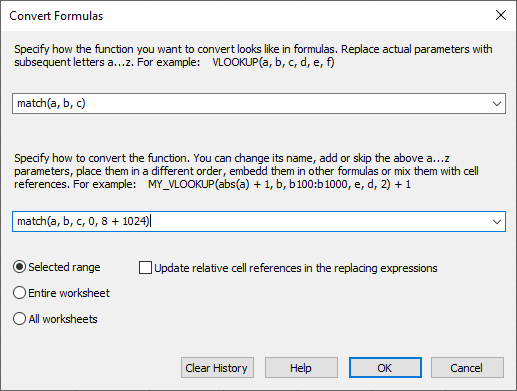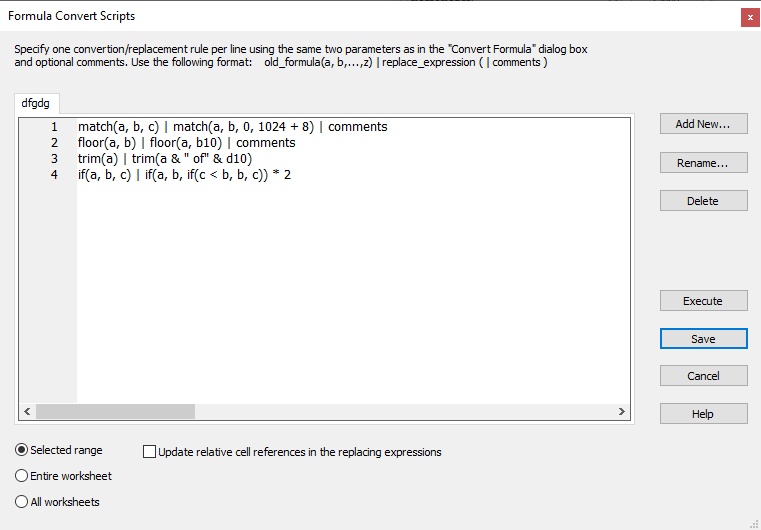Replacing formulas automatically in worksheets

To replace any function(s) in all your formulas instantly, use the "Tools > Convert Function in Formulas" command.

You can easily replace a given function by any other functions or expressions that uses some or all of the original parameters in any order, embed them in any other functions or expressions, mix this with cell references
or constant values etc.

In the first edit field you need to specify how the function look like in formulas.
Replace actual parameters with subsequent letters a...z. In real formulas in your worksheet these parameters can be any expressions and nested functions, for example:

• VLOOKUP(a, b, c, d, e, f)
• TRIM(a)
• IF(a, b, c)
• MATCH(a, b, c)

In the second edit field you must specify the expression that will replace that function, for example:

MY_VLOOKUP(abs(a) + 1, b, b100:b1000, e, d, 2) + 1
• TRIM(a & " of" & d10)
• IF(a, b, IF(c < b, b, c)) * 2
• MATCH(a, b, 0, 1, 1)

After clicking "OK", all found formulas will be updated. You can specify whether these changes are to be
performed for the current selection only, for the entire worksheet or for all worksheets in the current workbook.
If the replacing expression includes new cell references and you want them to updated in subsequent cells same as when
e.g. when filling cells, check the "Update relative cell references" check box.
Updating is fast. Millions of formulas can be changed instantly.
GS-Calc displays information on how many formulas has been modified.

Besides changing GS-Calc worksheets you use this procedure to change Excel *.xlsx data.
For example, if you have an Excel worksheet using the new XMATCH or XLOOKUP Excel formula and have Excel 2019
that doesn't have these functions.

For example, to convert/replace

• XMATCH(a, b, c, d)

You can use:

• For vertical binary searching (where COLS(b)=1):

MATCH(a, b, c, IF((d)=2, 8, IF((f)=-2, 16, IF((c)=-1, 512, 0))))

• For the classic linear searching returning that last match:

MATCH(a, b, c, IF((c)=-1, 512, 0))

• For the classic linear searching:

MATCH(a, b, c)

To convert/replace

• XLOOKUP(a, b, c, d, e, f)

You can use a construction similar to the following one:

• For vertical binary searching (where COLS(b)=1):

INDEX(c, MATCH(a, b, e, IF((f)=2, 8, IF((f)=-1, 512, 256))), )

Note:

GS-Calc match(), vlookup() functions offer a much wider parameter selection and thus much better performance than the X- Excel counterparts.
For example, even if a given range is not sorted, you can still perform fast binary
searching if you specify
SEARCH::AutoSort (or 1024)
in the "search mode" parameters. GS-Calc will perform the required sorting internally
during the 1st update and will keep the sorting indices (an unlimited number of them)
up to date. This may results in thousands of times faster updating.
In addition to the "find first" or "find last" match modes you can also explicitly
specify which matching occurrence should be returned.To simplify performing multiple conversions, you can define scripts with various
conversion rules same as above. To do this, use the "Tools > Function Conversion Scripts"
command

Scripts are just named sequences of conversions. You can add any number of them and each one
can contain any number of conversion definitions.